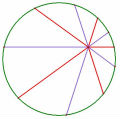# 101 宜蘭高中

 老王 老王發私訊 加為好友 目前離線 1# 大 中 小 發表於 2012-6-8 22:00  顯示全部帖子 推到噗浪 推到臉書 $$\displaystyle \sum _{k=1}^{10} \frac{k \times 2^k}{(k+1)(k+2)}$$ $$\displaystyle =\sum _{k=1}^{10} k \times 2^k \times (\frac{1}{k+1}-\frac{1}{k+2})$$ $$\displaystyle =1+\sum _{k=1}^9 \frac{1}{k+2}[(k+1) \times 2^{k+1}-k \times 2^k]-\frac{10 \times 2^{10}}{12}$$ $$\displaystyle =1+\sum _{k=1}^9 \frac{1}{k+2} \times 2^k \times (2k+2-k)-\frac{5 \times 2^9}{3}$$ $$\displaystyle =1+2+4+ \cdots +512-\frac{2560}{3}$$ $$\displaystyle =\frac{509}{3}$$ [ 本帖最後由 老王 於 2012-6-8 10:03 PM 編輯 ] 名豈文章著官應老病休飄飄何所似Essential isolated singularity UID261 帖子308 閱讀權限10 上線時間943 小時 註冊時間2009-5-14 最後登入2014-3-17  查看詳細資料 TOP

TOP

## 回復 6# weiye 的帖子

$$\ P(6,-2),A(a,0),B(0,b),Q(-4,3)$$

TOP

﻿